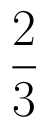Select Page

# Determinant CBSE Maths 12 Science MCQ Solutions in English

Determinant CBSE Maths 12 Science MCQ Solutions in English to enable students to get Solutions in a narrative video format for the specific question.

Expert Teacher provides Determinant CBSE Maths 12 Science MCQ Solutions through Video Solutions in English language. This video solution will be useful for students to understand how to write an answer in exam in order to score more marks. This teacher uses a narrative style for a question from Determinant not only to explain the proper method of answering question, but deriving right answer too.

Please find the question below and view the Solution in a narrative video format.

Question:

Solution Video in English:

You can select video Solutions from other languages also. Please check Solutions in ( Hindi )

## Similar Questions from CBSE, 12th Science, Maths, Determinant

Question 1 :  If A is a square matrix of order 3 and |A| = 5 then |3A| = ? (View Answer Video)

Question 2 : If the area of a triangle is 35 sq units with vertices (2, -6), (5, 4) and (k, 4). Then k is: (View Answer Video)

Question 3 : Evaluate the determinant:. (View Answer Video)

Question 5 : Evaluate  the determinants in :. (View Answer Video)

### Probability

Question 1 : In a school, there are 1,000 students, out of which 380 are girls. Out of 380 girls, 10% of the girls scored highest in GS. What is the probability that a student chosen randomly scored highest in GS given that the chosen student is a girl?  (View Answer Video)

Question 2 : If each element of a second-order determinant is either zero or one, what is the probability that the value of the determinant is positive?  (View Answer Video)

Question 3 :  A bag contains 3 red and 7 black balls. 2 balls are selected at random one by one without replacement. If the second selected ball happens to be red, what is the probability that the first selected ball is also red?   (View Answer Video)

Question 4 : A problem in mathematics is given to 4 students A, B, C, D. Their chances of solving the problem, respectively, areand. What is the probability that atmost one of them solve the problem?  (View Answer Video)

Question 5 : How many times must a man toss a fair coin, so that the probability of having at least one head is more than 80%.  (View Answer Video)

### Differential Equations

Question 1 : Write the degree of the differential equation(View Answer Video)

Question 2 : Write the sum of the order and degree of the differential equation(View Answer Video)

Question 3 : If x cos(a + y) = cos y, then prove thatHence show that(View Answer Video)

Question 4 : Find the particular solution of the differential equationgiven that y = 0, when x = 0. (View Answer Video)

Question 5 : Find the general solution of differential equation(View Answer Video)

### Linear Programming

Question 1 : The objective function is maximum or minimum, which lies on the boundary of the feasible region. (View Answer Video)